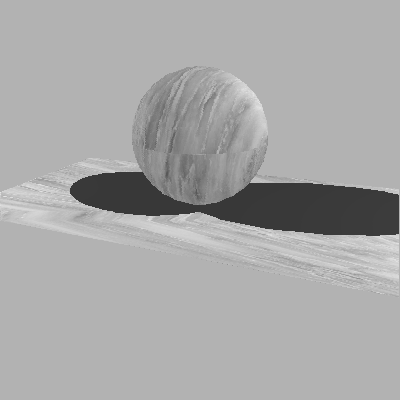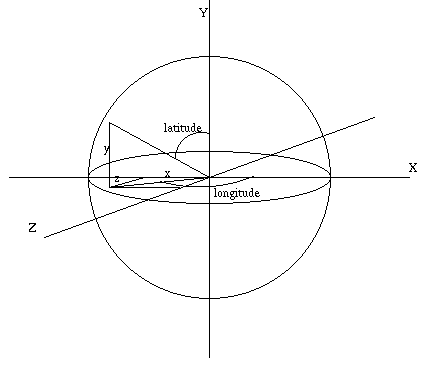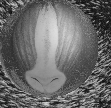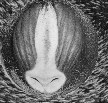# Combining Texture Mapping with Lighting Models

by Chris Bentley

## What is Texture Mapping?

Texture mapping is a method for pasting images onto arbitrary polygonal objects. This technique has become very popular because it allows surfaces to be covered with highly complex designs, without requiring a complex polygonal model. For example a simple model of a cube can have photographs of the interior of a room texture mapped onto its sides, thus creating the illusion of a highly complex architectural model.

In texture mapping, an image is used essentially as a look up table: whenever a pixel in the projection of the model is about to be plotted, a lookup is performed into the image. Whatever color is in the image at the sampled position, becomes the color that is plotted for the model. The color discovered by checking in the image can be used without modification, or it can be blended with the color computed from lighting equations for the model. It is also common for the value from the image to be used to modulate one of the parameters of the ordinary lighting equations. For example the surface normal at a point in the model can be perturbed proportional to the value found in the image lookup.Fig 1.Sphere texture mapped with marble

## Texture Coordinates

In texture mapping an association is formed between the vertices of a 3D polygonal model, and the pixels of an image. The image is typically viewed as existing in a normalized "image" or "texture" space where the coordinates, (u,v), run from 0.0 to 1.0. The parameter u is usually thought of as the distance along the width of the image, and v, is the distance along the height of the image. In other words: 0.0 <= u <= 1.0 , and 0.0 <= v <= 1.0 , and a (u,v) value of (0.5,0.5) corresponds to half way across the image and half way up, namely the center. Texture mapping, then, is a mapping from a 3D world coordinate system to a 2D image space: O(x,y,z) -> T(u,v).

## Texture Mapping Steps

Texture mapping has two primary steps:
1. (u,v) are associated with every vertex of polygonal model. These parameters "pin" the image to the object, as if the image were cloth.
2. Once the vertices have been assigned coordinates, (u,v) coordinates need to be interpolated for points in the interior of polygons.

Assigning (u,v) to Vertices

There are numerous methods, described by Watt [WATT92], for associating (u,v) values to each vertex. One simple method can be termed "latitude/logitude mapping". Another method assigns (u,v) values at the stage of building the polgon model of an object. If this is done, knowledge of the shape of the object can be used to make intelligent assignments of parameter values.

In latitude/longitude mapping, the normal of the surface at each vertex is used to calculate (u,v) values for that vertex. The normal is a vector of three components: N = (x,y,z). The question is how to represent this triple as a vector of only two components. The answer is to convert the 3D cartesian coordinates into spherical coordinates, i.e to longitude and latitude. Spherical coordinates can express any point on a unit sphere by two angles. The longitude angle describes how far "around" the sphere the point is, and the latitude angle describes how far "up".Fig 2.Latitude/Longitude mapping

Since, we can think of the vertex normal as specifying a point on a unit sphere, we can convert each vertex normal N = (x,y,z), to spherical coordinates, and then use the latutude/longitude tuple as (u,v) values for indexing into our texture image.

Each point, P on the unit sphere, can be expressed in spherical coordinates as:

```          longitude = arctan( z/x );
latitude = arccos( y );
```
The (u,v) values calculated in this manner can then be used to index into the texture image. For example a u value of 0.3 would translate to an image pixel one third of the way across the image.

## Implementating Latitude/Longitude mapping

```/****************************************************************/
/* norm_uv - convert normal vector to u, v coordinates          */
/****************************************************************/
static void norm_uv( norm, u, v )
VECT norm;
double *u;
double *v;
{
*u = (FLT_ZERO(norm)) ? 0.5 : atan2pi(norm, norm);
*v = (asinpi(norm) + 0.5);
}

/****************************************************************/
/* map - find image color for a given u, v pair                 */
/****************************************************************/
static unsigned char map( u, v, image )
double u;
double v;
IMAGE *image;
{
unsigned int x, y, index;

x = (unsigned int)(u * image->width);
y = (unsigned int)(v * image->height);

index = (y * image->width) + x;

return( image->data[index] );

}
```

## Interpolating (u,v) Values for Pixels Within Polygon

Once the vertices of a model have been assigned (u,v) texture coordinates, there still remains the task of interpolating these coordinates to derive (u,v) values for pixels in the interior of the polygon. This can be done using bilinear interpolation, much like the interpolation of intensity values done in Gouraud shading. This method suffers from the same problem as scan line shading algorithms in general: the (u,v) are interpolated uniformly in screen space. However, because of perspective distortion, the point half way between two vertices in screen space is not half way between the vertices in world space! So uniform interpolation is not in fact correct in this setting.

In Gouraud shading, when rendering a scan line, only z and intensity values are interpolated because along the scan line y is constant. In texture mapping, as a scan line is rendered, both u and v need to be incremented for each pixel, because in general a horizontal line across the projected polygon will translate to a diagonal path taken through the texture space. As can be seen in Fig 3. below, as the indicated scan line is rendered pixel by pixel, both the u and v will need to be interpolated.Fig 3.Texture scan line rendering

## Combining Texture Mapping with Lighting

Texture mapping can "paste" an image onto any faceted object. The texture image is used to lookup what color to plot for any pixel in the rendered object. However, it is also possible to blend the color from the texture lookup with the color calculated via lighting equations. Similarly, the texture color can be used to modulated the ambient, diffuse or specular components of the light reflecting from the object. Fig 4. below shows the texture color blended with the lighting color, with a weight of 0.4 for the texture color, while Fig 5. gives the texture component a weight of 0.8.Fig 4. Blending texture color and lightingFig 5. Texture color weighted more heavily

## Conclusion

There are many areas of advanced texture mapping that are not explored here: mip-mapping and area sampling to reduce anti-aliasing; perspective correction; other mapping techniques. Texture mapping is a very powerful method for adding photographically realistic effects to computer generated images, without the expense of creating excessively complex polygonal models.

## ExamplesFig 6.Teapot texture mapped with marble

## References

[WATT92]
Watt, Alan, and Watt, Mark, "Advanced Animation and Rendering Techniques", Addison Wesley, 1992

Chris Lawson Bentley
chrisb@wpi.edu
Fri Apr 28 14:54:17 EDT 1995